# Vibration Analysis of Rotating Machinery

This example shows how to analyze vibration signals from a gearbox using time-synchronous averaging and envelope spectra. These functions are especially useful in the predictive maintenance of gearboxes, which contain multiple rotating components: gears, shafts and bearings.

This example generates and analyzes vibration data for a gearbox whose shafts rotate at a fixed speed. Time-synchronous averaging is used to isolate vibration components associated with a specific shaft or gear and average out all other components. Envelope spectra are especially useful in identifying localized bearing faults that cause high-frequency impacts.Consider an idealized gearbox that consists of a 13-tooth pinion meshing with a 35-tooth gear. The pinion is coupled to an input shaft connected to a prime mover. The gear is connected to an output shaft. The shafts are supported by roller bearings on the gearbox housing. Two accelerometers, ${\mathrm{A}}_{1\text{\hspace{0.17em}}}$and ${\mathrm{A}}_{2}$, are placed on the bearing and gearbox housings, respectively. The accelerometers operate at a sample rate of 20 kHz.

The pinion rotates at a rate ${\mathit{f}}_{\mathrm{Pinion}}$ = 22.5 Hz or 1350 rpm. The rotating speed of the gear and output shaft is

${\mathit{f}}_{\mathrm{Gear}}={\mathit{f}}_{\mathrm{Pinion}}×\frac{\mathrm{Number}\text{\hspace{0.17em}}\mathrm{of}\text{\hspace{0.17em}}\mathrm{pinion}\text{\hspace{0.17em}}\mathrm{teeth}\text{\hspace{0.17em}}\left({\mathit{N}}_{\mathit{p}}\right)}{\mathrm{Number}\text{\hspace{0.17em}}\mathrm{of}\text{\hspace{0.17em}}\mathrm{gear}\text{\hspace{0.17em}}\mathrm{teeth}\text{\hspace{0.17em}}\left({\mathit{N}}_{\mathit{g}\text{\hspace{0.17em}}}\right)}$ .

The tooth-mesh frequency, also called gear-mesh frequency, is the rate at which gear and pinion teeth periodically engage:

${\mathit{f}}_{\mathrm{Mesh}}=\text{\hspace{0.17em}}{\mathit{f}}_{\mathrm{Pinion}}×{\mathit{N}}_{\mathit{p}\text{\hspace{0.17em}}}=\text{\hspace{0.17em}}{\mathit{f}}_{\mathrm{Gear}}×{\mathit{N}}_{\mathit{g}}$ .

```fs = 20E3; % Sample Rate (Hz) Np = 13; % Number of teeth on pinion Ng = 35; % Number of teeth on gear fPin = 22.5; % Pinion (Input) shaft frequency (Hz) fGear = fPin*Np/Ng; % Gear (Output) shaft frequency (Hz) fMesh = fPin*Np; % Gear Mesh frequency (Hz)```

Generate vibration waveforms for the pinion and the gear. Model the vibrations as sinusoids occurring at primary shaft gear mesh frequencies. Analyze 20 seconds of vibration data.

The gear-mesh waveform is responsible for transmitting load and thus possesses the highest vibration amplitude. ${\mathrm{A}}_{2}$ records vibration contributions from the two shafts and the gear-mesh. For this experiment, the contributions of the bearing rolling elements to the vibration signals recorded by ${\mathrm{A}}_{2}$ are considered negligible. Visualize a section of noise-free vibration signal.

```t = 0:1/fs:20-1/fs; vfIn = 0.4*sin(2*pi*fPin*t); % Pinion waveform vfOut = 0.2*sin(2*pi*fGear*t); % Gear waveform vMesh = sin(2*pi*fMesh*t); % Gear-mesh waveform plot(t, vfIn + vfOut + vMesh) xlim([0 0.25]) xlabel('Time (s)') ylabel('Acceleration')```### Generate High-Frequency Impacts Caused by a Local Fault on a Gear Tooth

Assume that one of the teeth of the gear is suffering from a local fault such as a spall. This results in a high-frequency impact occurring once per rotation of the gear.The local fault causes an impact that has a duration shorter than the duration of tooth mesh. A dent on the tooth surface of the gear generates high-frequency oscillations over the duration of the impact. The frequency of impact is dependent on gearbox component properties and its natural frequencies. In this example, it is arbitrarily assumed that the impact causes a 2 kHz vibration signal and occurs over a duration of about 8% of `1/fMesh`, or 0.25 milliseconds. The impact repeats once per rotation of the gear.

```ipf = fGear; fImpact = 2000; tImpact = 0:1/fs:2.5e-4-1/fs; xImpact = sin(2*pi*fImpact*tImpact)/3;```

Make the impact periodic by convolving it with a comb function.

```xComb = zeros(size(t)); Ind = (0.25*fs/fMesh):(fs/ipf):length(t); Ind = round(Ind); xComb(Ind) = 1; xPer = 2*conv(xComb,xImpact,'same'); ```

Add the fault signal `xPer` to the shaft signal. Add white Gaussian noise to the output signals for both the fault-free and the faulty gear to model the output from ${\mathrm{A}}_{2\text{\hspace{0.17em}}}$.

```vNoFault = vfIn + vfOut + vMesh; vFault = vNoFault + xPer; vNoFaultNoisy = vNoFault + randn(size(t))/5; vFaultNoisy = vFault + randn(size(t))/5; ```

Visualize a segment of the time history. The impact locations are indicated on the plot for the faulty gear by the inverted red triangles. They are almost indistinguishable.

```subplot(2,1,1) plot(t,vNoFaultNoisy) xlabel('Time (s)') ylabel('Acceleration') xlim([0.0 0.3]) ylim([-2.5 2.5]) title('Noisy Signal for Healthy Gear') subplot(2,1,2) plot(t,vFaultNoisy) xlabel('Time (s)') ylabel('Acceleration') xlim([0.0 0.3]) ylim([-2.5 2.5]) title('Noisy Signal for Faulty Gear') hold on MarkX = t(Ind(1:3)); MarkY = 2.5; plot(MarkX,MarkY,'rv','MarkerFaceColor','red') hold off```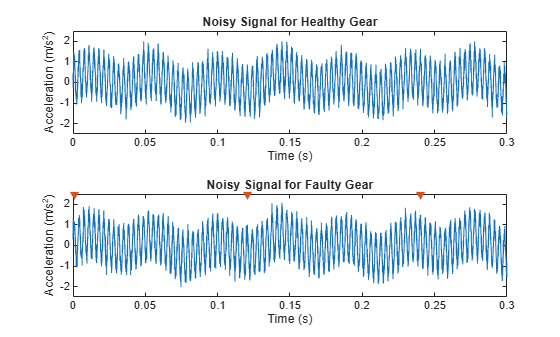### Compare Power Spectra for Both Signals

Localized tooth faults cause distributed sidebands to appear in the neighborhood of the gear mesh frequency:

`${\mathit{f}}_{\mathrm{s}\mathrm{ide}\mathrm{b}\mathrm{and},\mathrm{Pinion}}={\mathit{f}}_{\mathrm{Mesh}}\text{\hspace{0.17em}}±\mathit{m}×{\mathit{f}}_{\mathrm{Pinion}}\text{\hspace{0.17em}\hspace{0.17em}\hspace{0.17em}\hspace{0.17em}\hspace{0.17em}\hspace{0.17em}\hspace{0.17em}\hspace{0.17em}\hspace{0.17em}\hspace{0.17em}\hspace{0.17em}}\forall \text{\hspace{0.17em}}\mathit{m}\in \left\{1,\text{\hspace{0.17em}}2,\text{\hspace{0.17em}}3,..\right\}$`

`${\mathit{f}}_{\mathrm{s}\mathrm{ide}\mathrm{b}\mathrm{and},\mathrm{Gear}}={\mathit{f}}_{\mathrm{Mesh}}\text{\hspace{0.17em}}±\mathit{m}×{\mathit{f}}_{\mathrm{Gear}}\text{\hspace{0.17em}\hspace{0.17em}\hspace{0.17em}\hspace{0.17em}\hspace{0.17em}\hspace{0.17em}\hspace{0.17em}\hspace{0.17em}\hspace{0.17em}\hspace{0.17em}\hspace{0.17em}}\forall \text{\hspace{0.17em}}\mathit{m}\in \left\{1,\text{\hspace{0.17em}}2,\text{\hspace{0.17em}}3,..\right\}$`

Calculate the spectrum of the healthy and faulty gears. Specify a frequency range that includes the shaft frequencies at 8.35 Hz and 22.5 Hz and the gear-mesh frequency at 292.5 Hz.

`[Spect,f] = pspectrum([vFaultNoisy' vNoFaultNoisy'],fs,'FrequencyResolution',0.2,'FrequencyLimits',[0 500]);`

Plot the spectra. Because the fault is on the gear and not the pinion, sidebands are expected to appear at ${\mathit{f}}_{\mathrm{s}\mathrm{ide}\mathrm{b}\mathrm{and},\mathrm{Gear}}$ and spaced ${\mathit{f}}_{\mathrm{Gear}}$ apart on the spectra. The spectra show the expected peaks at `fGear`, `fPin`, and `fMesh`. However, the presence of noise in the signal makes the sideband peaks at ${\mathit{f}}_{\mathrm{s}\mathrm{ide}\mathrm{b}\mathrm{and},\mathrm{Gear}}$ indistinguishable.

```figure plot(f,10*log10(Spect(:,1)),f,10*log10(Spect(:,2)),':') xlabel('Frequency (Hz)') ylabel('Power Spectrum (dB)') hold on plot(fGear,0,'rv','MarkerFaceColor','red') plot(fPin,0,'gv','MarkerFaceColor','green') plot(fMesh,0,'bv','MarkerFaceColor','blue') hold off legend('Faulty','Healthy','f_{Gear}','f_{Pinion}','f_{Mesh}')```Zoom in on the neighborhood of the gear-mesh frequency. Create a grid of gear and pinion sidebands at ${\mathit{f}}_{\mathrm{s}\mathrm{ide}\mathrm{b}\mathrm{and},\mathrm{Gear}}$ and ${\mathit{f}}_{\mathrm{s}\mathrm{ide}\mathrm{b}\mathrm{and},\mathrm{Pinion}}$.

```figure p1 = plot(f,10*log10(Spect(:,1))); xlabel('Frequency (Hz)') ylabel('Power Spectrum (dB)') xlim([250 340]) ylim([-70 -40]) hold on p2 = plot(f,10*log10(Spect(:,2))); harmonics = -5:5; SBandsGear = (fMesh+fGear.*harmonics); [X1,Y1] = meshgrid(SBandsGear,ylim); SBandsPinion = (fMesh+fPin.*harmonics); [X2,Y2] = meshgrid(SBandsPinion,ylim); p3 = plot(X1,Y1,':r'); p4 = plot(X2,Y2,':k'); hold off legend([p1 p2 p3(1) p4(1)],{'Faulty Gear';'Healthy Gear';'f_{sideband,Gear}';'f_{sideband,Pinion}'})```It is not clear if the peaks align with the gear sidebands ${\mathit{f}}_{\mathrm{s}\mathrm{ide}\mathrm{b}\mathrm{and},\mathrm{Gear}}$.

### Apply Time-Synchronous Averaging to the Output Vibration Signal

Note that it is difficult to separate the peaks at the gear sidebands, ${\mathit{f}}_{\mathrm{SideBand},\text{\hspace{0.17em}}\mathrm{Gear}}$, and the pinion sidebands, ${\mathit{f}}_{\mathrm{SideBand},\text{\hspace{0.17em}}\mathrm{Pinion}}$. The previous section demonstrated difficulty in separating peaks and determining if the pinion or the gear is affected by faults. Time-synchronous averaging averages out zero-mean random noise and any waveforms not associated with frequencies of the particular shaft. This makes the process of fault detection easier.

Use the function `tsa` to generate time-synchronized waveforms for both the pinion and the gear.

Specify time-synchronized pulses for the pinion. Calculate the time-synchronous average for 10 rotations of the pinion.

```tPulseIn = 0:1/fPin:max(t); taPin = tsa(vFaultNoisy,fs,tPulseIn,'NumRotations',10);```

Specify time-synchronized pulses for the gear. Calculate the time-synchronous average for 10 rotations of the gear.

```tPulseOut = 0:1/fGear:max(t); taGear = tsa(vFaultNoisy,fs,tPulseOut,'NumRotations',10);```

Visualize the time-synchronized signals for a single rotation. The impact is comparatively easier to see on the time-synchronous averaged signal for the gear, while it is averaged out for the pinion shaft. The location of the impact, indicated on the plot with a marker, has a higher amplitude than neighboring gear-mesh peaks.

`The tsa` function without output arguments plots the time-synchronous average signal and the time-domain signals corresponding to each signal segment in the current figure.

```figure subplot(2,1,1) tsa(vFaultNoisy,fs,tPulseIn,'NumRotations',10) xlim([0.5 1.5]) ylim([-2 2]) title('TSA Signal for Pinion') subplot(2,1,2) tsa(vFaultNoisy,fs,tPulseOut,'NumRotations',10) xlim([0.5 1.5]) ylim([-2 2]) title('TSA Signal for Gear') hold on plot(1.006,2,'rv','MarkerFaceColor','red') hold off```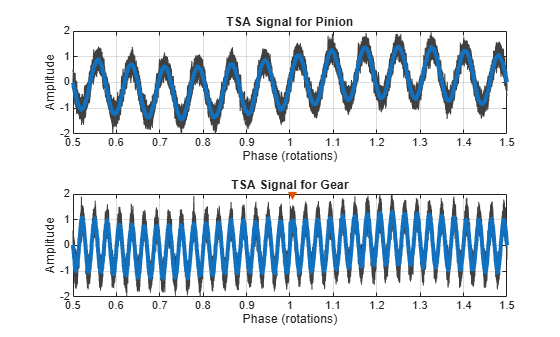### Visualize the Power Spectra for Time-Synchronous Averaged Signals

Calculate the power spectrum of the time-synchronous averaged gear signal. Specify a frequency range that covers 15 gear sidebands on either side of the gear mesh frequency of 292.5 Hz. Notice the peaks at ${\mathit{f}}_{\mathrm{s}\mathrm{ide}\mathrm{b}\mathrm{and},\mathrm{Gear}}$.

```figure pspectrum(taGear,fs,'FrequencyResolution',2.2,'FrequencyLimits',[200 400]) harmonics = -15:15; SBandsGear=(fMesh+fGear.*harmonics); [X1,Y1] = meshgrid(SBandsGear,ylim); [XM,YM] = meshgrid(fMesh,ylim); hold on plot(XM,YM,'--k',X1,Y1,':r') legend('Power Spectra','Gear-Mesh Frequency','f_{sideband,Gear}') hold off title('TSA Gear (Output Shaft)')```Visualize the power spectra of the time-synchronous averaged pinion signal in the same frequency range. This time, plot grid lines at ${\mathit{f}}_{\mathrm{s}\mathrm{ide}\mathrm{b}\mathrm{and},\mathrm{Pinion}}$ frequency locations.

```figure pspectrum(taPin,fs,'FrequencyResolution',5.8,'FrequencyLimits',[200 400]) SBandsPinion = (fMesh+fPin.*harmonics); [X2,Y2] = meshgrid(SBandsPinion,ylim); [XM,YM] = meshgrid(fMesh,ylim); hold on plot(XM,YM,'--b',X2,Y2,':k') legend('Power Spectra','Gear-Mesh Frequency','f_{sideband,Pinion}') hold off title('TSA Pinion (Input Shaft)')```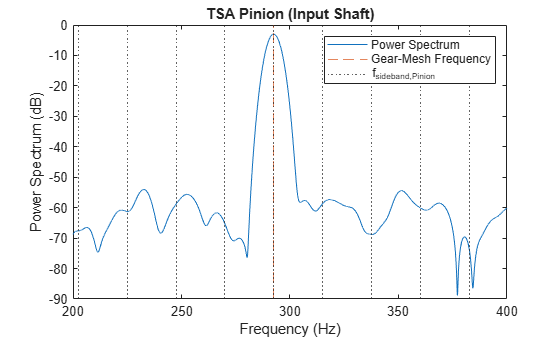Notice the absence of prominent peaks at ${\mathit{f}}_{\mathrm{s}\mathrm{ide}\mathrm{b}\mathrm{and},\mathrm{Pinion}}$ in the plot.

The power spectra of the original signal contains waveforms from two different shafts, as well as noise. It is difficult to distinguish the sideband harmonics. However, observe the prominent peaks at the sideband locations on the spectrum of the time-synchronous averaged gear signal. Also observe the nonuniformity in sideband magnitudes, which are an indicator of localized faults on the gear. On the other hand, sideband peaks are absent from the spectrum of the time-synchronous averaged pinion signal. This helps us conclude that the pinion is potentially healthy.

By averaging out the waveforms that are not relevant, the `tsa` function helps identify the faulty gear by looking at sideband harmonics. This functionality is especially useful when it is desirable to extract a vibration signal corresponding to a single shaft, from a gearbox with multiple shafts and gears.

### Add a Distributed Fault in the Pinion and Incorporate its Effects into the Vibration Signal

A distributed gear fault, such as eccentricity or gear misalignment , causes higher-level sidebands that are narrowly grouped around integer multiples of the gear-mesh frequency.To simulate a distributed fault, introduce three sideband components of decreasing amplitude on either side of the gear-mesh frequency.

```SideBands = -3:3; SideBandAmp = [0.02 0.1 0.4 0 0.4 0.1 0.02]; % Sideband amplitudes SideBandFreq = fMesh + SideBands*fPin; % Sideband frequencies vSideBands = SideBandAmp*sin(2*pi*SideBandFreq'.*t);```

Add the sideband signals to the vibration signal. This results in amplitude modulation.

`vPinFaultNoisy = vFaultNoisy + vSideBands;`

Visualize a section of the time history for the gearbox affected by the distributed fault.

```plot(t,vPinFaultNoisy) xlim([0.6 0.85]) xlabel('Time (s)') ylabel('Acceleration') title('Effects of Sideband Modulation')```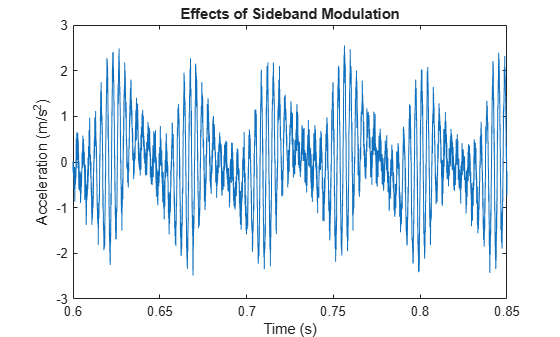Recompute the time-synchronous averaged signal for the pinion and the gear.

```taPin = tsa(vPinFaultNoisy,fs,tPulseIn,'NumRotations',10); taGear = tsa(vFaultNoisy,fs,tPulseOut,'NumRotations',10); ```

Visualize the power spectrum of the time-synchronous averaged signal. The three sidebands in the time-synchronous averaged signal of the pinion are more pronounced which indicate the presence of distributed faults. However, the spectrum of the time-synchronous averaged gear signal remains unchanged.

```subplot(2,1,1) pspectrum(taPin,fs,'FrequencyResolution',5.8,'FrequencyLimits',[200 400]) hold on plot(X2,Y2,':k') legend('Power Spectrum','f_{sideband,Pinion}','Location','south') hold off title ('TSA Pinion (Input Shaft)') subplot(2,1,2) pspectrum(taGear,fs,'FrequencyResolution',2.2,'FrequencyLimits',[200 400]) hold on plot(X1,Y1,':r') legend('Power Spectrum','f_{sideband,Gear}') hold off title ('TSA Gear (Output Shaft)')```In conclusion, the `tsa` function helps extract the gear and pinion contributions from the overall vibration signal. This in turn helps identify the specific components that are affected by localized and distributed faults.

### Vibration Analysis of Rolling Element Bearing Faults

Localized faults in a rolling element bearing may occur in the outer race, the inner race, the cage, or a rolling element. Each of these faults is characterized by its own frequency, which is usually listed by the manufacturer or calculated from the bearing specifications. An impact from a localized fault generates high-frequency vibrations in the gearbox structure between the bearing and response transducer . Assume that the gears in the gearbox are healthy and that one of the bearings supporting the pinion shaft is affected by a localized fault in the inner race. Neglect the effects of radial load in the analysis.The bearing, with a pitch diameter of 12 cm, has eight rolling elements. Each rolling element has a diameter of 2 cm. The angle of contact $\theta$ is ${15}^{\circ }$. It is common practice to place the accelerometer on a bearing-housing while analyzing bearing vibration. Acceleration measurements are recorded by${\mathrm{A}}_{1}$, an accelerometer located on the faulty bearing housing.

Define the parameters for the bearing.

```n = 8; % Number of rolling element bearings d = 0.02; % Diameter of rolling elements p = 0.12; % Pitch diameter of bearing thetaDeg = 15; % Contact angle in degrees```

The impacts occur whenever a rolling element passes the localized fault on the inner race. The rate at which this happens is the ball pass frequency-inner race (BPFI). The BPFI can be calculated using

${\mathit{f}}_{\mathrm{BPFI}}\text{\hspace{0.17em}}=\frac{\mathit{n}{\text{\hspace{0.17em}}×\text{\hspace{0.17em}}\mathit{f}}_{\mathrm{Pin}\text{\hspace{0.17em}}}}{2\text{\hspace{0.17em}}}\left(1+\frac{\mathit{d}}{\mathit{p}}\mathrm{cos}\text{\hspace{0.17em}}\theta \text{\hspace{0.17em}}\right)$.

`bpfi = n*fPin/2*(1 + d/p*cosd(thetaDeg))`
```bpfi = 104.4889 ```

Model each impact as a 3 kHz sinusoid windowed by a Kaiser window. The defect causes a series of 5-millisecond impacts on the bearing. Impulses in the early stages of pits and spalls cover a wide frequency range up to about 100 kHz .

```fImpact = 3000; tImpact = 0:1/fs:5e-3-1/fs; xImpact = sin(2*pi*fImpact*tImpact).*kaiser(length(tImpact),40)';```

Make the impact periodic by convolving it with a comb function. Since ${\mathrm{A}}_{1}$ is closer to the bearing, adjust the amplitude of the impact such that it is prominent with respect to the gearbox vibration signal recorded by ${\mathrm{A}}_{2}$.

```xComb = zeros(size(t)); xComb(1:round(fs/bpfi):end) = 1; xBper = 0.33*conv(xComb,xImpact,'same');```

Visualize the impact signal.

```figure plot(t,xBper) xlim([0 0.05]) xlabel('Time (s)') ylabel('Acceleration') title('Impacts Due to Local Fault on the Inner Race of the Bearing')```Add the periodic bearing fault to the vibration signal from the healthy gearbox.

```vNoBFaultNoisy = vNoFault + randn(size(t))/5; vBFaultNoisy = xBper + vNoFault + randn(size(t))/5;```

Compute the spectra of the signals. Visualize the spectrum at lower frequencies. Create a grid of the first ten BPFI harmonics.

```pspectrum([vBFaultNoisy' vNoBFaultNoisy' ],fs,'FrequencyResolution',1,'FrequencyLimits',[0 10*bpfi]) legend('Damaged','Healthy') title('Bearing Vibration Spectra') grid off harmImpact = (0:10)*bpfi; [X,Y] = meshgrid(harmImpact,ylim); hold on plot(X/1000,Y,':k') legend('Healthy','Damaged','BPFI harmonics') xlim([0 10*bpfi]/1000) hold off```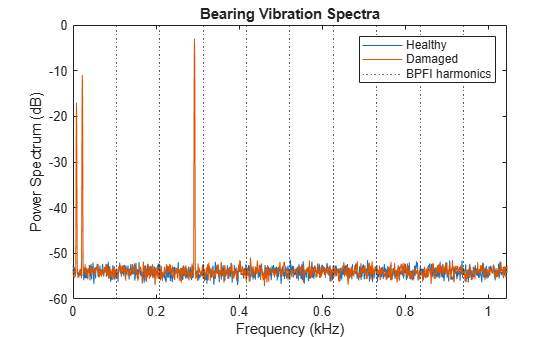At the lower end of the spectrum, the shaft and mesh frequencies and their orders obscure other features. The spectrum of the healthy bearing and the spectrum of the damaged bearing are indistinguishable. This flaw highlights the necessity for an approach that can isolate bearing faults.

BPFI is dependent on the ratio $\mathit{d}/\mathit{p}$ and the cosine of the contact angle $\theta$. An irrational expression for BPFI implies that bearing impacts are not synchronous with an integer number of shaft rotations. The `tsa` function is not useful in this case because it averages out the impacts. The impacts do not lie on the same location in every averaged segment.

The function `envspectrum` (envelope spectrum) performs amplitude demodulation, and is useful in extracting information about high-frequency impacts.

Compute and plot the envelope signals and their spectra. Compare the envelope spectra for the signals with and without the bearing fault. Visualize the spectrum at lower frequencies. Create a grid of the first ten BPFI harmonics.

```figure envspectrum([vNoBFaultNoisy' vBFaultNoisy'],fs) xlim([0 10*bpfi]/1000) [X,Y] = meshgrid(harmImpact,ylim); hold on plot(X/1000,Y,':k') legend('Healthy','Damaged','BPFI harmonics') xlim([0 10*bpfi]/1000) hold off```Observe that BPFI peaks are not prominent in the envelope spectrum because the signal is polluted by noise. Recall that performing `tsa` to average out noise is not useful for bearing-fault analysis because it also averages out the impact signals.

The `envspectrum` function offers a built-in filter that can be used to remove noise outside the band of interest. Apply a bandpass filter of order 200 centered at 3.125 kHz and 4.167 kHz wide.

```Fc = 3125; BW = 4167; envspectrum([vNoBFaultNoisy' vBFaultNoisy'],fs,'Method','hilbert','FilterOrder',200,'Band',[Fc-BW/2 Fc+BW/2]) harmImpact = (0:10)*bpfi; [X,Y] = meshgrid(harmImpact,ylim); hold on plot(X/1000,Y,':k') legend('Healthy','Damaged','BPFI harmonics') xlim([0 10*bpfi]/1000) hold off```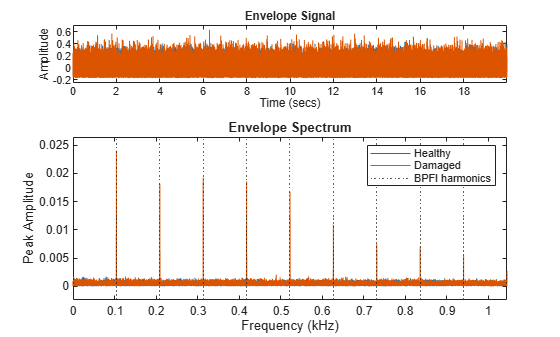The envelope spectrum effectively brings in the passband content to baseband, and therefore shows the presence of prominent peaks at the BPFI harmonics below 1 kHz. This helps conclude that the inner race of the bearing is potentially damaged.

In this case, the frequency spectrum of the faulty bearing clearly shows BPFI harmonics modulated by the impact frequency. Visualize this phenomenon in the spectra, close to the impact frequency of 3 kHz.

```figure pspectrum([vBFaultNoisy' vNoBFaultNoisy'],fs,'FrequencyResolution',1,'FrequencyLimits',(bpfi*[-10 10]+fImpact)) legend('Damaged','Healthy') title('Bearing Vibration Spectra')```Observe that the separation in frequency between peaks is equal to BPFI.

### Conclusions

This example used time-synchronous averaging to separate vibration signals associated with both a pinion and a gear. In addition, `tsa` also attenuated random noise. In cases of fluctuating speed (and load ), order tracking can be used as a precursor to `tsa` to resample the signal in terms of shaft rotation angle. Time-synchronous averaging is also used in experimental conditions to attenuate the effects of small changes in shaft speed.

Broadband frequency analysis may be used as a good starting point in the fault analysis of bearings . However, its usefulness is limited when the spectra in the neighborhood of bearing impact frequencies contain contributions from other components, such as higher harmonics of gear-mesh frequencies in a gearbox. Envelope analysis is useful under such circumstances. The function `envspectrum` can be used to extract envelope signals and spectra for faulty bearings, as an indicator of bearing wear and damage.

### References

1. Scheffer, Cornelius, and Paresh Girdhar. Practical Machinery Vibration Analysis and Predictive Maintenance. Amsterdam: Elsevier, 2004.

2. Randall, Robert Bond. Vibration Based Condition Monitoring: Industrial, Aerospace and Automotive Applications. Chichester, UK: John Wiley and Sons, 2011.

3. Lacey, S. J. An Overview of Bearing Vibration Analysis. (From: http://www.maintenanceonline.co.uk/maintenanceonline/content_images/p32-42%20Lacey%20paper%20M&AM.pdf)

4. Brandt, Anders. Noise and Vibration Analysis: Signal Analysis and Experimental Procedures. Chichester, UK: John Wiley and Sons, 2011.